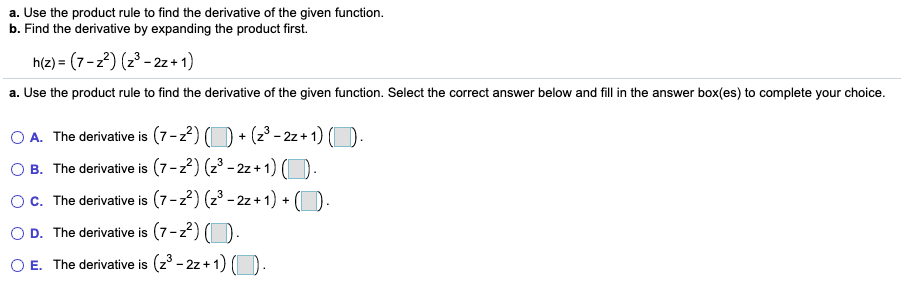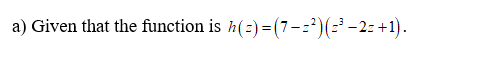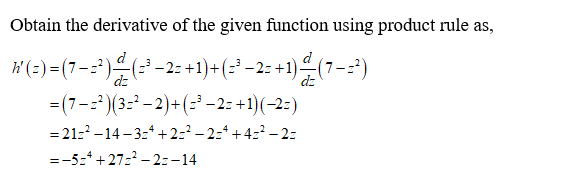# a. Use the product rule to find the derivative of the given function.b. Find the derivative by expanding the product first.h(z) = (7-z2) (z3 - 2z + 1)a. Use the product rule to find the derivative of the given function. Select the correct answer below and fill in the answer box(es) to complete your choice.O A. The derivative is (7-z2)B. The derivative is (7-2) (23 - 2z+ 1) O.c. The derivative is (7-22) (23 - 2z + 1) + (D+ (z° - 2z + 1) (O.D. The derivative is (7-2) (D.E. The derivative is (z° - 2z + 1) (O.

Question
4 viewshelp_outlineImage Transcriptionclosea. Use the product rule to find the derivative of the given function. b. Find the derivative by expanding the product first. h(z) = (7-z2) (z3 - 2z + 1) a. Use the product rule to find the derivative of the given function. Select the correct answer below and fill in the answer box(es) to complete your choice. O A. The derivative is (7-z2) B. The derivative is (7-2) (23 - 2z+ 1) O. c. The derivative is (7-22) (23 - 2z + 1) + (D + (z° - 2z + 1) (O. D. The derivative is (7-2) (D. E. The derivative is (z° - 2z + 1) (O. fullscreen
check_circle

Step 1Step 2...

### Want to see the full answer?

See Solution

#### Want to see this answer and more?

Solutions are written by subject experts who are available 24/7. Questions are typically answered within 1 hour.*

See Solution
*Response times may vary by subject and question.
Tagged in